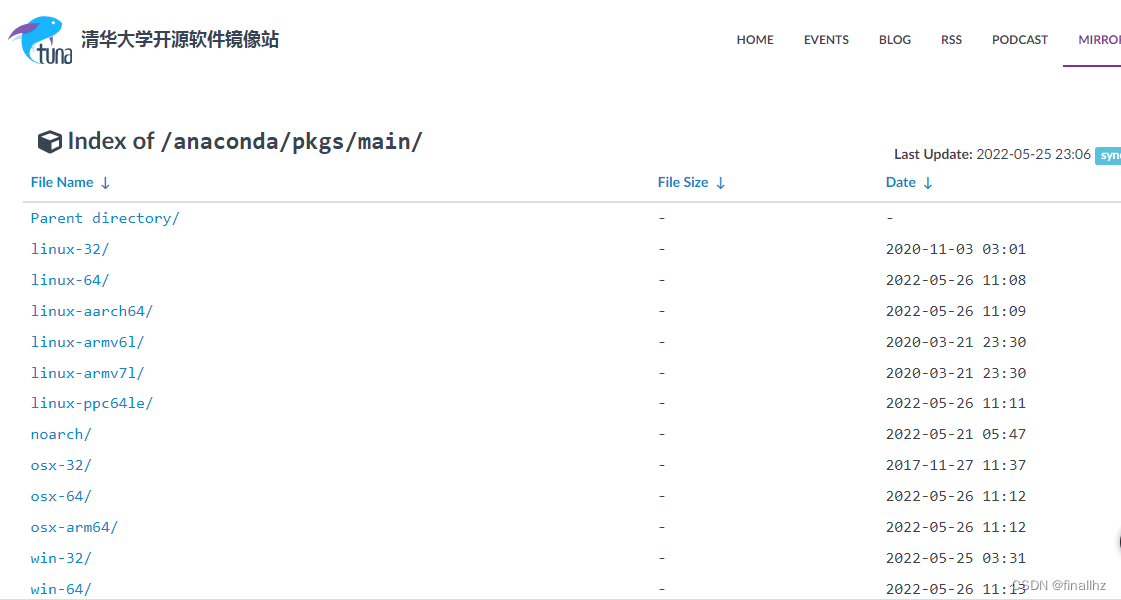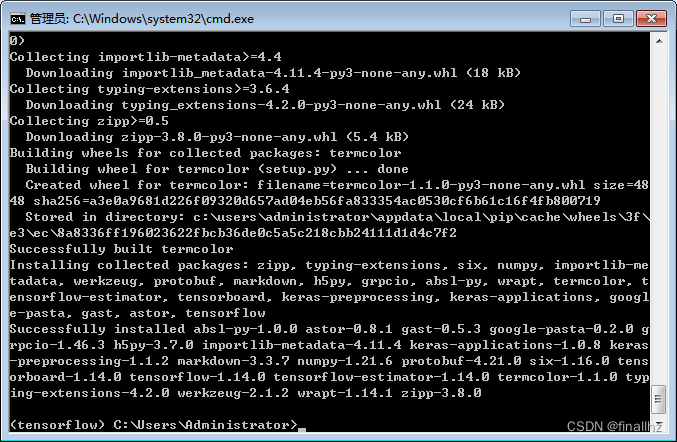# Numpy数组与矩阵（一）

## 1 ndarray对象

​ Numpy最重要的的一个特点是N为数组对象ndarray，它是一系列同类型数据的集合，以0 下标为开始对集合中的元素建立索引。

​ ndarray的组成部分有：

• 一个指向数据（内存或内存映射文件中的一块数据）的指针；
• 数据类型或dtype，描述在数组中的固定大小的格子；
• 一个表示数组形状（shape）的元组，表示各维度大小的元组；
• 一个跨度元组（stride），其中的整数指的是为了前进到当前维度的下一个元素需要"跨过"的字节数。

​ 创建一个ndarray只需要调用Numpy的array函数就可以了，它的语法结构如下所示：

``````numpy.array(object, dtype = None, copy = True, order = None, subok = False, ndmin = 0)
``````

​ 以下进行参数说明：

​ 下面我们给出几个实例：

``````
import numpy as np

a = np.array([1, 2, 3, 4, 5])

data = [[1, 2, 3, 4, 5], [6, 7, 8, 9, 10]]
b = np.array(data)
``````

​ 当我们需要查看数组的维度时，直接调用ndim属性就可以了，具体我们可以使用 变量名.ndim来查看。

## 2 数据类型

​ NumPy支持的数据类型比Python内置的类型要多很多，其中的不分类型也是对应于Python的内置类型而精心设计的。

​ 以下我将会举出几个实例：

``````import numpy as np
c = np.array([1, 2, 3, 4, 5])
print(c)
c.dtype
``````​ 运行以上程序，我们知道数组c中各元素的数据类型是int32。

``````d = np.array([-11.7, 41.55, 32.6, -19.88, 32.11, -9.5])
print(d)
d.dtype
``````## 3 数组属性

​ Numpy的数组中比较重要的ndarray对象属性如下表所示。

​ 我们通过实例来说明数组的这些特性。我们首先需要创建数组，然后直接调用数组的这些属性。

``````a = np.arange(25, dtype = np.float_).reshape(5, 5)
b = np.array([1, 2, 3, 4, 5])
print(a)
print(b)
``````​ 我们创建了a，b两个不同维度的数组，之后我们调用属性查看这两个数组之间的差别。

``````print('数组a的属性：')
print(a.ndim)
print(a.shape)
print(a.size)
print(a.dtype)
print(a.itemsize)
``````​ 以上就是数组a的所有属性显示。

``````print('数组b的属性：')
print(b.ndim)
print(b.shape)
print(b.size)
print(b.dtype)
print(b.itemsize)
``````​ 以上就是数组b的所有属性显示。

## 4 创建数组

​ 以下给出数组创建函数：

### 4.1 零元素数组

​ 我们创建全部都是由0来填充的数组，要使用zeros函数，下面将会给出具体创建函数以及参数说明。

``````numpy.zeros(shape, dtype = float, order = 'C')
``````

​ 参数说明如下：

​ 实例说明：

``````import numpy as np
a = np.zeros((5,2))
print(a)
``````### 4.2 一元素数组

​ 利用== ones函数==来实现全部由1来填充元素的数组，具体语法结构以及参数说明如下述：

``````numpy.ones(shape, dtype = None, order = 'C')
``````

​ 至于以上提到的参数，和零元素数组中的参数说明相同，在此不再概述。

​ 下面我们将举出实例进行说明：

``````import numpy as np
b = np.ones((5, 5))
print(b)
print(b.dtype)
``````### 4.3 arange函数

​ 在Numpy中，我们还可以通过arange函数来创建数组，并且用arange函数创建出来的是ndarray对象。具体的函数使用以及参数说明如下述：

``````numpy.arange(start, stop, step, dtype)
``````

​ 参数说明如下：

​ 实例演示：

``````import numpy as np
arr1 = np.arange(0, 10, 2)
arr2 = np.arange(10, -10, -4)
arr3 = np.arange(1, 5, 2, dtype = float)
arr4 = np.arange(10)
print('arr1 = ', arr1)
print('arr2 = ', arr2)
print('arr3 = ', arr3)
print('arr4 = ', arr4)
``````### 4.4 等差数列数组

​ Numpy中的linspace函数是用来创建一个一维数组，该数组是由一个等差数列构成的。具体语法结构如下所述：

``````nmupy.linspace(start, stop, num = , endpoint = True, retstep = Fasle, dytpe = None)
``````

​ 具体参数说明如下：

​ 实例说明：

``````import numpy as np
a = np.linspace(0, 5, 6)
b = np.linspace(0, 5, 6, endpoint = False)
print("a = ", a)
print("b = ", b)
``````### 4.5 等比数列数组

​ Numpy中的logspace函数可以用来创建一个等比数列，语法格式如下：

``````nmupy.logspace(start, stop, num = , endpoint = True, base = , dtype = None)
``````

​ 参数具体说明如下：

​ 具体实例说明：

``````import numpy as np
a = np.logspace(1, 10, 10 ,base = 2)
print(a)
``````## 5 数据索引与切片

​ ndarray对象可以通过索引过着切片进行访问和修改，Numpy切片语法和Python列表切片的方法相同。下面我我将为大家介绍数组的索引与切片。

### 5.1 索引

​ 我们将会了解如何通过索引和切片来选择元素，以此来获得其中的值。数组的索引总是使用"[]"来索引数组的元素，以便这些元素可以被单独引用，用于不同的用途，下面我们将通过案例来说明如何进行索引操作。

``````import numpy as np
a = np.random.randn(6)
print("a = ", a)
print("a = ", a)
print("a[-1] = ", a[-1])
``````​ 依据上面的例子，我们可以看到，在一维数组中，我们可以通过中括号指定索引第i个值（从0开始计数）。我们的例子中a，输出的是第三个数。

​ 当然，我们也可以从后向前索引， 最后一个元素的索引是-1，像例子中a[-1]，输出的就是最后一个数。

``````print("a[[1,4]] = ", a[[1,4]])
``````​ 如果我们需要选择多各项，可以在方括号中传递索引数组，就比如我们需要第2个和第5个数字，我们就可以使用a[[1, 4]]，来达到获取数组中的两个数据。

​ 当然，我们还可以定义一个二维数组，二维数组被表示成由行和列组成的矩形数组，由两个轴来定义，其中轴0表示行，轴1表示列。我们要是想通过索引来表示一个值，那么我们需要输入这个数字的行、列索引，行索引在前，列索引在后。具体方法是：数组名[行索引，列索引]。

​ 具体实例如下：

``````import numpy as np
b = np.random.randn(4, 4)
print("b = ", b)
print("b[3, 3] = ", b[3, 3])
``````### 5.2 切片

​ 切片在Python中的应用十分重要。切片的定义是在一个数组中，我们通过提取其中的一部分元素来形成一个新的数组。当使用Python列表对数组进行切片时，我们的得到数组是一个副本。为了获取某个数组的一个切片，我们通过以下方式：

``````arrays_name[start:stop:step]
``````

​ 上述方法表示，从索引start开始到索引stop（ 不包含），之间的步长是step。默认情况下，会分别设置为： start = 0stop = 维度的大小step = 1

​ 下面我们通过实例说明：

``````import numpy as np
a = np.arange(10)
print('a = ', a)
print('a[0:5] =', a[0: 5])
print('a[1:7:2]', a[1:7:2])
``````​ 对于二维数组，切片也同样适用，不同的是，行和列需要分别定义。示例如下：

``````import numpy as np
a = np.random.randn(24).reshape(6, 4)
print('a = ')
print(a)
print('获取数组a的第三行数据：')
print(a[2, :])
print('获取数组a的第一列数据：')
print(a[:, 0])
``````​ 对于二维数组还有很多有趣的操作，感兴趣的话，可以自己动手自我尝试一下。

Original: https://blog.csdn.net/gogo_monster/article/details/123398427
Author: 秋刀鱼的知更鸟
Title: Numpy数组与矩阵（一）

*
- 一、系统环境
- 二、安装步骤

## 一、系统环境

Python环境：Python3.7；conda 4.10.3

## 二、安装步骤

``````D:\anaconda\condabin
D:\anaconda\Scripts
D:\anaconda\Library\bin
D:\anaconda\
``````

1.如果先前配置过清华等镜像地址，建议首先执行

``````conda config --remove-key channels
``````

``````CondaKeyError: 'channels': key 'channels' is not in the config file
``````

``````show_channel_urls: true
``````

2.然后需要配置源

``````conda config --add channels http://mirrors.tuna.tsinghua.edu.cn/anaconda/cloud/msys2/win-64
conda config --set show_channel_urls yes
````````````show_channel_urls: true
channels:
- http://mirrors.tuna.tsinghua.edu.cn/anaconda/pkgs/main/win-64
- http://mirrors.tuna.tsinghua.edu.cn/anaconda/pkgs/free/win-64
- http://mirrors.tuna.tsinghua.edu.cn/anaconda/cloud/conda-forge/win-64
- http://mirrors.tuna.tsinghua.edu.cn/anaconda/cloud/msys2/win-64
``````

3.上面两步都是针对镜像源的配置，接下来才是TensorFlow的流程。首先要配置虚拟环境。

``````conda create -n
``````

4.安装完成后，需要激活虚拟环境，我这里是

``````activate tensorflow
``````

https://mirrors.tuna.tsinghua.edu.cn/pypi/web/simple/tensorflow/``````pip install D:\tensorflow-1.14.0-cp37-cp37m-win_amd64.whl
``````Original: https://blog.csdn.net/weixin_48289706/article/details/124981481
Author: finallhz
Title: conda安装指定版本TensorFlow